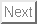Previous: Ensembles

## Microstates

Imagine we have counters, blue on one side and green on the other, and we toss them and place them on a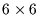checkerboard. Full information involves listing the colour at each site: this is the equivalent of a microstate.

Many different patterns are possible, such as the following. Every configuration is equally likely--or unlikely--to occur: There are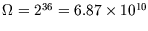patterns and the the probability of each is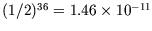. (This satisfies the postulate of equal a priori probabilities''.)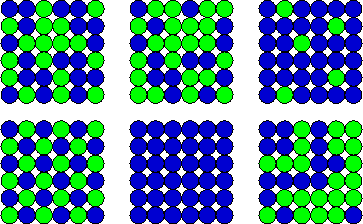Suppose from a distance we only knew how many counters were green and how many blue, without being able to distinguish different arrangements of the same numbers of counters. Then a macrostate'' would be characterised simply by the total number of green counters (the rest being blue).

Clearly, most macrostates correspond to many microstates. If the macroscopic description is 15 green'', the following are a few of the allowed microstates: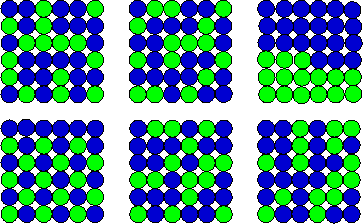How many are there in total? This is the common problem of splitting a group of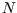into two smaller groups, of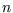and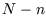, without caring about the ordering in each group, and the number of ways of doing it is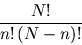Think of counters on squares: there are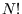ways of puttingdistinguishable counters onsquares. However ifof the counters are green, there are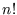ways of arranging the green counters among themselves without changing the pattern, and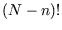ways of arranging the blues.
Here,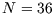and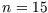, so the total is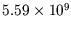. For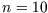there are only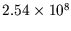, whereas for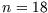, there are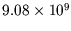. This is the maximum.

The numbers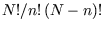are called the binomial coefficients (since they enter the binomial expansion) and they are written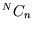or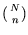.Previous: Ensembles
Judith McGovern 2004-03-17